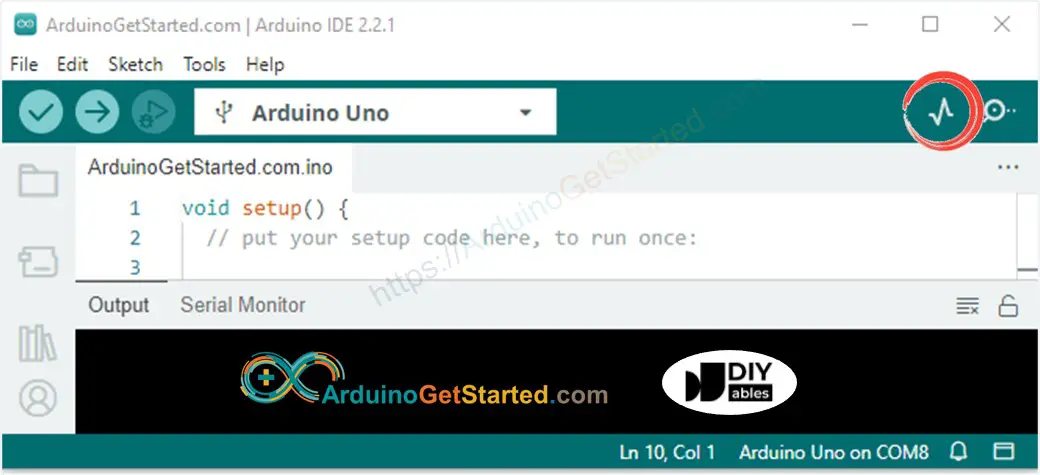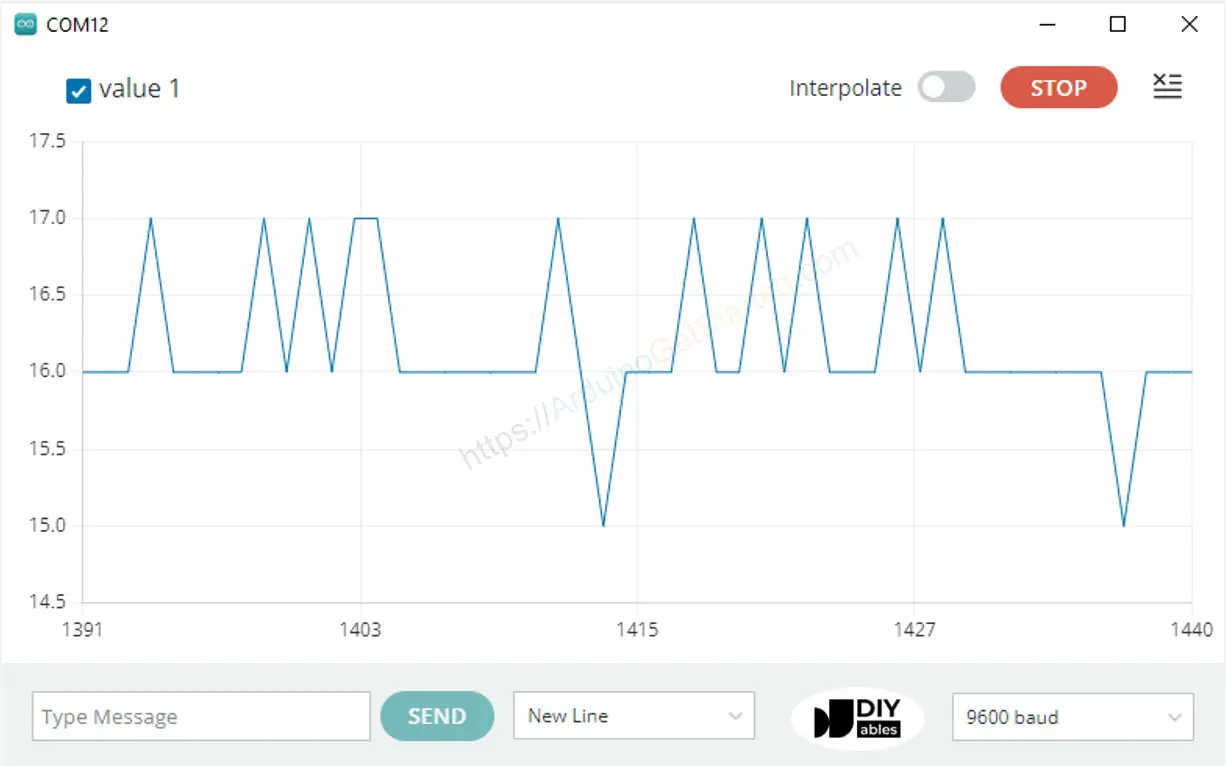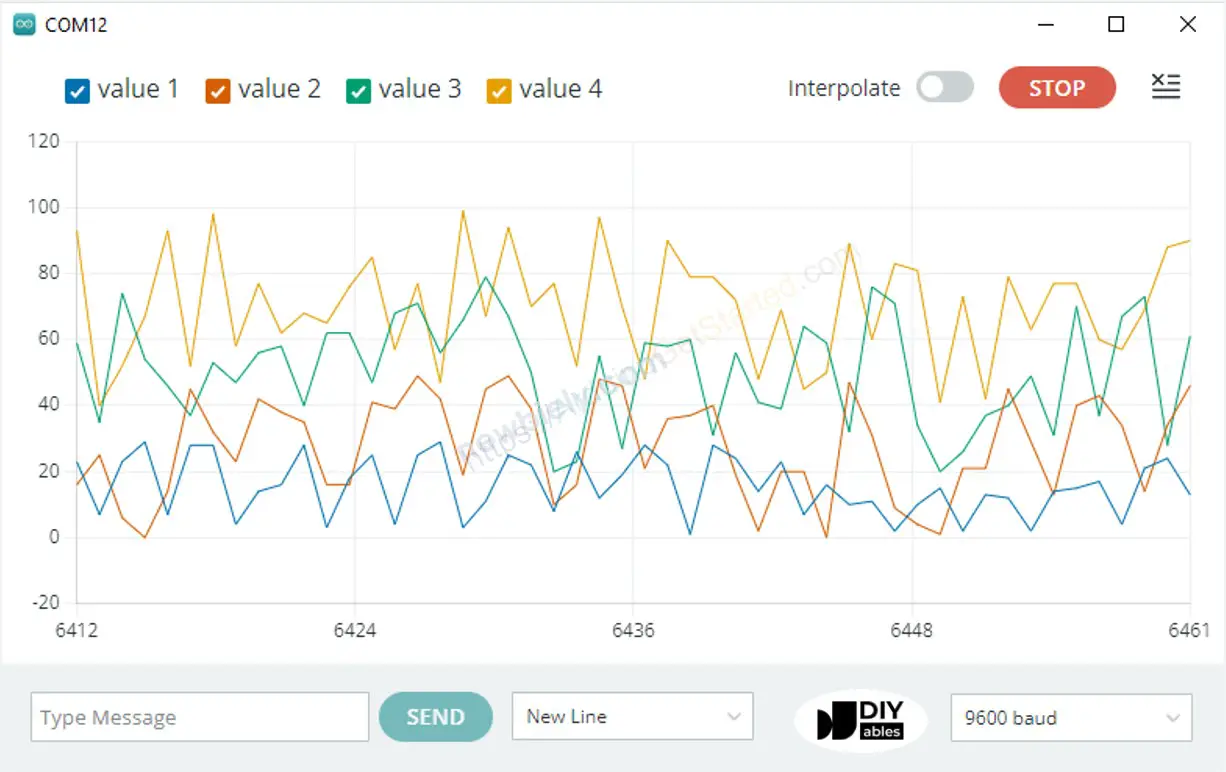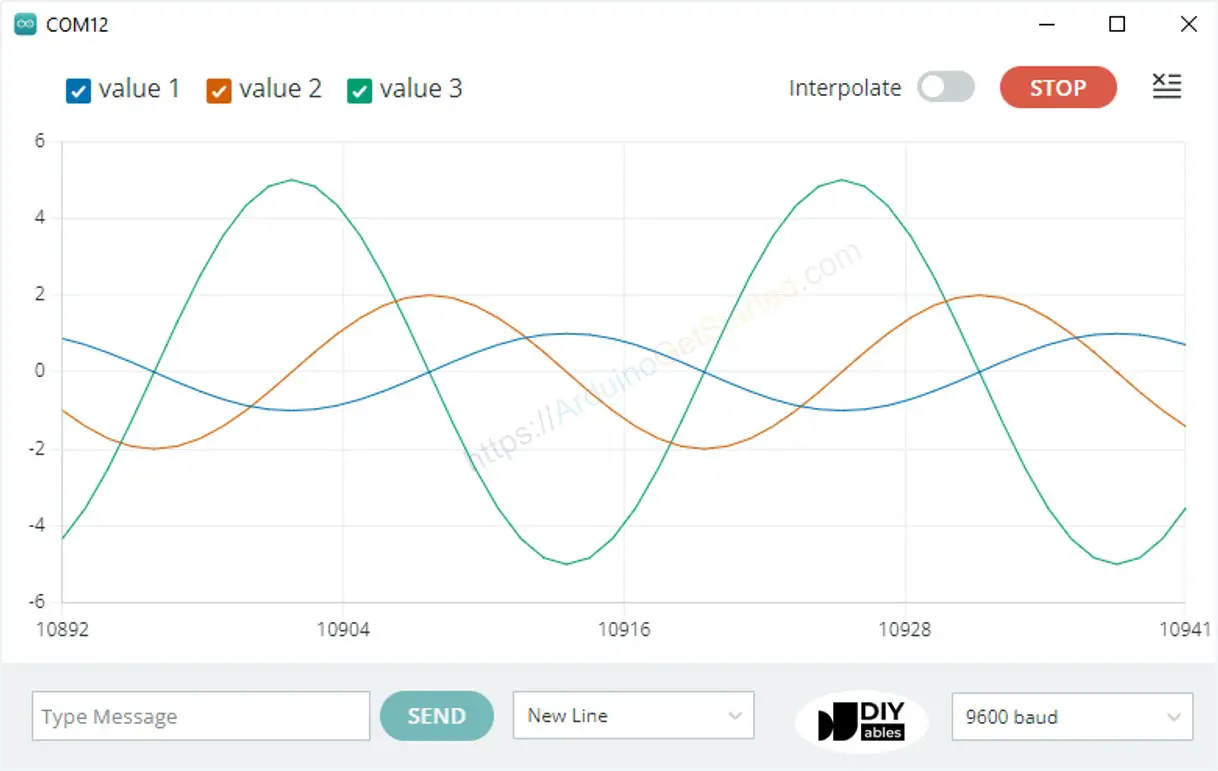# Arduino - Serial Plotter

## Hardware Required

Or you can buy the following sensor kit:

Please note: These are affiliate links. If you buy the components through these links, We may get a commission at no extra cost to you. We appreciate it.

Serial Plotter is one of the tools in Arduino IDE. Arduino can read the temperature, humidity or any kind of sensor data, and send it to Serial Plotter. Serial Plotter receives data from Arduino and visualizes data as waveforms. Serial Plotter can visualize not only single but also multiple sensor data in the same graph.

Data is exchanged between Serial Plotter and Arduino via USB cable, which is also used to upload the code to Arduino. Therefore, To use Serial Plotter, we MUST connect Arduino and PC via this cable.

Serial Plotter includes a selection box to select the serial baud rate and a graph:

• X-axis: represent the time. It has 500 points. The time between each point is the time between two consecutive Serial.println() function calls. This time is usually equal to the time of loop() function.
• Y-axis: represents the values received from Arduino. The Y-axis automatically adjusts itself as the value increases or decreases.

## How To Open Serial Plotter

On Arduino IDE, Go to Tools Serial Plotter## Plotting of Single Line in Graph

To print a single graph, we just need to send the data and terminate it by “\r\n” character.

In detail, we just need to use Serial.println() function

Serial.println(variable);

※ NOTE THAT:

Serial.println() automatically appends “\r\n” characters after data.

### Example Code

/* * Created by ArduinoGetStarted.com * * This example code is in the public domain * * Tutorial page: https://arduinogetstarted.com/tutorials/arduino-serial-plotter */ void setup() { Serial.begin(9600); } void loop() { int y1 = analogRead(A0); Serial.println(y1); delay(100); }

#### Quick Steps

• Copy the above code and open with Arduino IDE
• Click Upload button on Arduino IDE to upload code to Arduino
• Open Serial Plotter
• Select baurate 9600
• See graph on Serial Plotter## Plotting of Multiple Lines in Graph

When we want to plot multiple variables, we need to separate variables from each other by “\t” or " " character. The last value MUST be terminated by “\r\n” characters.

In detail:

• The first variable
Serial.print(variable_first);
• The middle variables
Serial.print("\t"); // or Serial.print(" ") Serial.print(variable_nth);
• The last variable
Serial.print("\t"); // or Serial.print(" ") Serial.println(variable_last);

### Example Code

/* * Created by ArduinoGetStarted.com * * This example code is in the public domain * * Tutorial page: https://arduinogetstarted.com/tutorials/arduino-serial-plotter */ void setup() { Serial.begin(9600); } void loop() { int y1 = analogRead(A0); int y2 = analogRead(A1); int y3 = analogRead(A2); int y4 = analogRead(A3); Serial.print(y1); Serial.print(" "); // a space ' ' or tab '\t' character is printed between the two values. Serial.print(y2); Serial.print(" "); // a space ' ' or tab '\t' character is printed between the two values. Serial.print(y3); Serial.print(" "); // a space ' ' or tab '\t' character is printed between the two values. Serial.println(y4); // the last value is followed by a carriage return and a newline characters. delay(100); }

Multiple Graph:### Example of 3 Sine Waveforms

/* * Created by ArduinoGetStarted.com * * This example code is in the public domain * * Tutorial page: https://arduinogetstarted.com/tutorials/arduino-serial-plotter */ void setup() { Serial.begin(9600); } void loop() { for(int i = 0; i < 360; i += 5) { float y1 = 1 * sin(i * M_PI / 180); float y2 = 2 * sin((i + 90)* M_PI / 180); float y3 = 5 * sin((i + 180)* M_PI / 180); Serial.print(y1); Serial.print("\t"); // a space ' ' or tab '\t' character is printed between the two values. Serial.print(y2); Serial.print("\t"); // a space ' ' or tab '\t' character is printed between the two values. Serial.println(y3); // the last value is followed by a carriage return and a newline characters. delay(100); } }

Multiple Sine Waveform Graph:## Video Tutorial

We are considering to make the video tutorials. If you think the video tutorials are essential, please subscribe to our YouTube channel to give us motivation for making the videos.